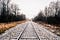Photo by Atharva Tulsi on Unsplash

# Explaining least-squares

## The basic linear regression model

As we start with machine learning, for me, the first model to understand is the least squares. A simple model that is easy to perform and gives a lot of insights about your datasets.

This model assumes that the expected E(Y|X) values of the dependent variable are linear to the inputs X1,…, Xn.

# Least Squares

It’s a supervised learning algorithm that takes an input vector, X^T = (X1, X2, …, Xp), and want to predict the output Y. The mathematical expression has the form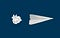Photo by Matt Ridley on Unsplash

# Machine learning project checklist

## Structuring Machine Learning Projects

When we build any type of project, there are checks that the project should accomplish. In the case of machine learning projects are nos distinct.

Since now we have been explaining the mathematics(Statistics, Probability, Linear algebra, Calculus) that will allow us to understand how machine learning models work. But machine learning is more than an algorithm, it’s easy to train a model by itself, the difficult part is making it useful!

# A basic structure for your machine learning projects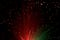Photo by Mihály Köles on Unsplash

# Separating Hyperplanes for classification

## The origins of Deep Learning and Support Vector Machines

The separating hyperplanes procedure constructs linear decision boundaries that explicitly try to separate the data into different classes as well as possible. With them, we will define the Support Vector Classifier.

Sometimes LDA and logistic regression explained in the previous post make avoidable errors, this can be solved using the following methods.

# Rosenblatt's Perceptron

This algorithm is the predecessor of the modern Deep learning advances, it tries to find a separating hyperplane by minimizing the distance of misclassified points to the decision boundary. The objective is to minimize the following function: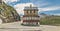Photo by Tim Trad on Unsplash

# The basic Methods for classification

## Linear methods for classification

As we try to classificate our data into distinct groups, our predictor G(x) takes values in a discrete set ζ and we can divide the input space into a collection of regions labeled according to the classification. With linear methods, we mean that the decision boundaries between our predicted classes are linear.

# Linear regression of an Indicator Matrix

All the response categories have an indicator variable. Thus if ζ has K classes, there will be K such indicators Yk: k = 1,…,k with Yk=1 if G=K, else 0, these are called dummy variables. …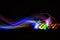Photo by Ryan Stone on Unsplash

# Explaining Subset Selection and Regularization Methods for Least-Squares Part-2

## Tuning the basic linear regression model

In previous posts, we introduced least-squares and explained some subset selection techniques. Today we are here to introduce more subset selection and shrinkage models.

# Least angle regression(LAR)

Least angle regression is a kind of forward stepwise that only enters as much of a predictor as it deserves. At the first step, it identifies the variable mos correlated with the response.

LAR adjusts the coefficient of a variable until another variable catches up in terms of correlation with the residual. Then the second variable is added and the coefficients are adjusted again. We repeat the process until all the variables are in the model.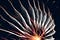Photo by Sebastian Kanczok on Unsplash

# Subset selection models applied

## Comparing distinct linear regression subset selection methods Ridge, Lasso, and Enet applied.

In the last two posts, we have been explaining the theory of linear regression and some subset selection techniques. It’s been very math-focused, but now we know all that we need to apply them using python.

To know how this application works, don’t miss the last two posts:

# Introducing the python libraries and the dataset

This example will use a dataset widely know between all data scientists and data scientist aspirants. First, we need to import the libraries that we will be using:

`import numpy as np import pandas as pd from itertools import cycle import matplotlib.pyplot…`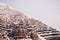Photo by Greg Rakozy on Unsplash

# Explaining subset selection and regularization methods for linear-squares

## Tuning the basic linear regression model

In the last post, we explained the most used linear regression machine learning technique, the least-squares. We explained distinct approaches to multiple linear regressions and regressions with multiple outputs.

But we assumed that we use all variables in the regression, today we will explain some techniques to select only a subset of variables. We do that because of two reasons:

# Reasons to use a subset selection

As we explained in the last post, the least-squares model minimizes the bias of the data, but not the variance. Here is where the bias-variance trade-off enters the game. Estimating a model, the expected prediction error at point x is: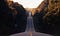Photo by Matt Duncan on Unsplash

# Integration in elementary terms

## Formulas to accelerate integration

In this post, we will summarise some of the most useful techniques to calculate integrals. This will allow you to avoid the limit notation, but there will always be some difficult integrals.

# Primitive functions

A function F satisfying F’ = f is called a primitive of f. Of course, a continuous function f always has a primitive.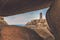Photo by Anthony Cantin on Unsplash

# The Fundamental theorem of calculus

## The relation between derivatives and integrals

The first theorem relates derivation with the integration of functions, it is divided between two theorems, let’s explain them.

# The first fundamental theorem of calculus

Let f be integrable on [a,b], and define F on [a,b] byPhoto by Max van den Oetelaar on Unsplash

# Integrals

## The area under the functions

After defining derivatives, we introduce the integrals. Not easy to define, by now we can understand them as the area between the function and the x-axis.

# Integral of a bounded region

First, we will define the integrals for bounded regions, assigning the integral of f on [a, b] to the area R(f, a, b). In this example, we use it for an always positive interval, but it is defined for negative and intervals having positive and negative values.

In the next gif, we show how to apply the idea, [a,b] is divided into subintervals, then the minimum(m_i) and maximum(M_i) value of the function for each…## Adrià Serra

Data scientst, this account will share my blog post about statistic, probability, machine learning and deep learming. #100daysofML